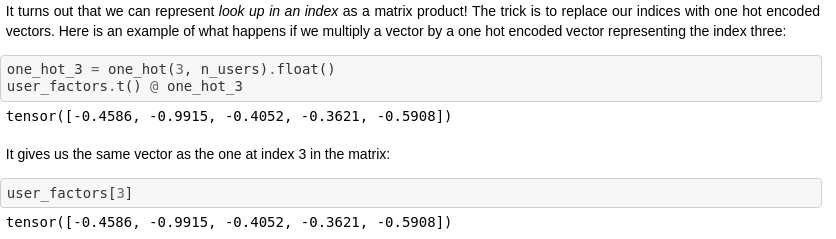# fast.ai 2020 — Lesson 6

`learn = cnn_learner(dls, resnet34, metrics=error_rate)learn.fine_tune(2, base_lr=0.1)`
`learn.fit_one_cycle(12, lr_max=slice(1e-6, 1e-4))`
`learn = cnn_learner(dls, resnet50, metrics=error_rate).to_fp16()`
`a = list(string.ascii_lowercase)a, len(a)CONSOLE: ('a', 26)def f1(o): return o+'a'def f2(o): return o+'b'dss = Datasets(a, [[f1,f2]])CONSOLE: ('aab',)dss = Datasets(a, [[f1],[f2]])CONSOLE: ('aa', 'ab')`
`def say_hello(name, say_what="Hello"): return f"{say_what} {name}."say_hello('Jeremy'),say_hello('Jeremy', 'Ahoy!')CONSOLE: ('Hello Jeremy.', 'Ahoy! Jeremy.')f = partial(say_hello, say_what="Bonjour")f("Jeremy"),f("Sylvain")CONSOLE: ('Bonjour Jeremy.', 'Bonjour Sylvain.')`Embedding is the same as matrix multiplication with a one hot encoded vector
`class Collab(Module):    def __init__(self, n_users, n_movies, n_factors, y_range=(0,5.5)):        self.user_factors = Embedding(n_users, n_factors)        self.user_bias = Embedding(n_users, 1)        self.movie_factors = Embedding(n_movies, n_factors)        self.movie_bias = Embedding(n_movies, 1)        self.y_range = y_range            def forward(self, x):        users = self.user_factors(x[:,0])        movies = self.movie_factors(x[:,1])        res = (users * movies).sum(dim=1, keepdim=True)        res += self.user_bias(x[:,0]) + self.movie_bias(x[:,1])        return sigmoid_range(res, *self.y_range)`

--

--